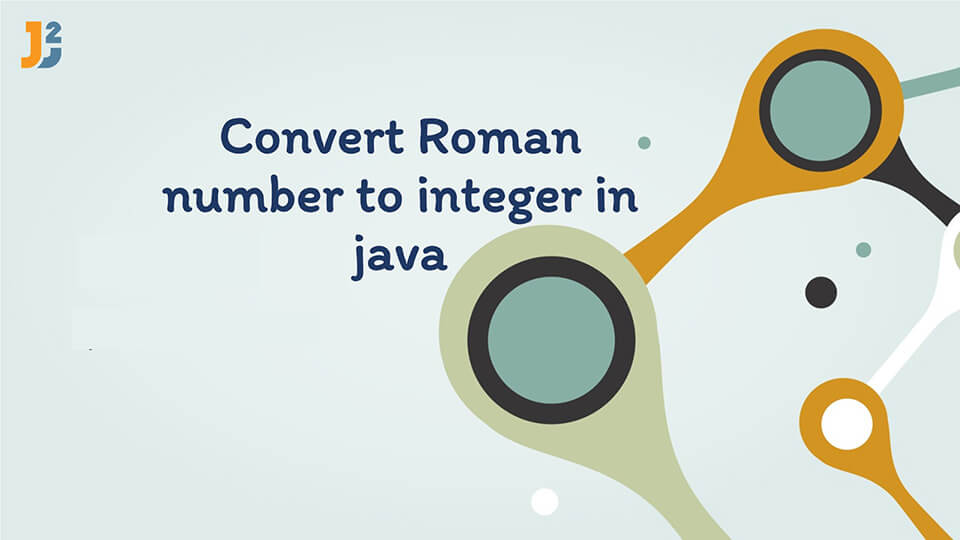# Convert Roman Number to Integer in JavaIn this article, we look into an interesting problem: `How to convert Roman Number to Integer in Java`.

Roman numerals are represented by seven different symbols : I, V, X, L, C, D, M. The Integral values associated with each symbol is shown in the table below:

Usually, roman numbers are written from left to right, which makes it easier for us to scan each roman character and add the corresponding value for each character to get the result.

`For Example:` Roman Number `III` has Integral value is 3 adding value of three I’s together (1+1+1=3).

Similarly for Roman Number: `DCLXVI` , Its integer value is : 500 + 100 + 50 + 10 +5 + 1= 666.

However, the Roman Number for 4 and 9 are not written as `IIII` and `VIIII`, respectively. They are written as IV which is (5-1 = 4), and for 9 it is `IX` (10 -1=9). This case is for letter `I`. There are 2 more cases to consider:

• `X` can be placed before `L` (50) and C (100), like `XL` and `XC` to make 40 and 90 respectively.
• Similarly, `C` can be placed before `D` and `M`, like `CD` and `CM` to make 400 and 900, respectively.

Hence, for Roman Number ‘CDXLIX’ the integer is 449.

`Note:` If a Roman Symbol having smaller value precedes a Symbol with Higher Value we need to subtract the higher value from the smaller value to get the result. Roman Numbers can have maximum Integral value: 3999 represented as : MMMCMXCIX.

## Implementation

• We will take a HashMap and for each Roman Character, we will put its respective value in the Map as shown in the table above. The Key would be the Roman Symbol and the Value will be the Integral Value of the Symbol.
• We iterate through each character in the given Number ,For each character we need to consider two cases :
• `Case 1 :` If the Character’s value is greater than the previous character value i.e. The condition is : `if (Value(charAt(i)) >Value(charAt(i-1))`, we need to perform subtraction as : `Value(charAt(i)) - 2* Value(charAt(i-1)) `; then add the result to our answer.
• `Case 2:` If the Character’s value is lesser than previous one we simply add its corresponding value to our answer and continue the process.
• In Case 1, we subtract twice the Previous Character value because we might have already added the value while processing it so we subtract twice the value.

Now let us have a quick look at the code in Java:

`Output:`

`Time Complexity :` The time complexity of this code is `O(n)`, where n is the length of Roman Number in String format as we are traversing String only once.

So that’s all about how to convert Roman Number to Integer in Java.

import_contacts

### You may also like:## Related Posts

•08 May

### Star Pattern programs in Java

Learn about how to print different star pattern in java.

•30 December

### Rock Paper Scissors Game in Java

Learn about how to implement Rock Paper Scissors game in java.

•25 December

### Find automorphic number in java

Learn about how to find automorphic number in java using different methods

•25 December

### Find nth prime number in java

Learn about how to find nth prime number in java.

•06 October

### Implement distance formula in java

In this post, we will see how to implement distance formula between two points in java. Formula to find distance between two points ( x1, y1) and (x2 , y2) is d= sqrt( (x2-x1)^2+ (y2 – y1)^2) Here is simple program to calculate distance between two points in java. [crayon-6096da931d1ec717176335/] Here we have used math.sqrt […]

•06 October

### Convert decimal to binary in java

In this post, we will see how to convert decimal to binary in java. There are lot of ways to convert decimal to binary in java.Let’s explore them one by one. Using Integer.toBinaryString() method You can simply use inbuilt Integer.toBinaryString() method to convert a decimal [crayon-6096da931d38d805236260/] Output: Binary representation of 12 : 1100 Binary representation […]

## Subscribe to our newletter

Get quality tutorials to your inbox. Subscribe now.